2013-04-10 14:51:37 mlkiller 阅读数 4225
• ###### MATLAB图像处理

全面系统的学习MATLAB在图像处理中的应用

20258 人正在学习 去看看 魏伟

# 一含义

模糊（平滑）是一种常用的图片处理方式，它的作用可以用来降低噪声，还有其他用途

看一下opencv 里面的公式g(i,j)是目标坐标的像素值， f(i+k,j+l)是k,l这些地方的像素值， h(k,l)是 kernel,  我不知道怎么去准确翻译它的意义，它是过滤器的系数。

简单的按照我的思路去理解，就是一个权值，模糊的含义是将所有的像素按照一定的权值进行运算，得到一个比较均衡的结果。

# 三 算法

1 均值模糊
均值模糊很简单就是周边所有的影响都是1，求平均值即可2 高斯模糊# 四均值模糊的代码和效果

先放上均值模糊的代码
void boxblur(Mat input ,Mat &out, int x, int y)
{
// accept only char type matrices
CV_Assert(input.depth() != sizeof(uchar));

out.create(input.size(),input.type());

int nChannels = input.channels();
int nRows = input.rows;
int nCols = input.cols;

int size = x * y;
float kernel = 1.0/size;

int i,j;
uchar* p;
uchar* q;
uchar R,G,B;

for( i = x; i < nRows - x; ++i)
{
q = out.ptr<uchar>(i);
for ( j = y; j < nCols - y; ++j)
{
float sumR = 0;
float sumG = 0;
float sumB = 0;
for (int k =0; k<x;k++)
{
p = input.ptr<uchar>(i-x+k);
for(int l = 0; l < y;l++)
{
sumB += input.at<uchar>(i - x + k,(j + l - y)*nChannels) * kernel;//p[(l + j -y)*nChannels ] * kernel;
sumG += input.at<uchar>(i - x + k,(j + l - y)*nChannels + 1) * kernel;//p[(l + j -y)*nChannels + 1] * kernel;
sumR += input.at<uchar>(i - x + k,(j + l - y)*nChannels + 2) * kernel;//p[(l + j -y)*nChannels + 2] * kernel;
}
}
q[j*nChannels] = sumB;
q[j*nChannels+1] = sumG;
q[j*nChannels+2] = sumR;
}
}

}原始 opencv 本文# 五高斯模糊的代码和效果

void gaussblur(Mat input ,Mat &out, int x, int y)
{
float sigma = 1.5;
Mat kernel;
float pi = 3.1415926;

kernel.create(x ,y ,CV_32F);

float mx = x/2.0;
float my = y/2.0;


       //这里有问题，后面做修正。
for (int i =0; i< x;i++)
{
for (int j =0; j<y;j++)
{
kernel.at<float>(i,j) = exp(-1 * ((i - mx) * (i - mx) +(j - my) * (j-my) )/( 2 * sigma * sigma))/(2 * pi * sigma *sigma) ;
}
}


int nChannels = input.channels();
int nRows = input.rows;
int nCols = input.cols;

out.create(input.size(),input.type());
uchar* p;
uchar* q;
float* s;

for(int  i = x; i < nRows - x; ++i)
{
q = out.ptr<uchar>(i);
for (int j = y; j < nCols - y; ++j)
{
float sumR = 0;
float sumG = 0;
float sumB = 0;
for (int k =0; k<x;k++)
{
p = input.ptr<uchar>(i-x+k);
s = kernel.ptr<float>(k);
for(int l = 0; l < y;l++)
{
sumB += p[(l + j -y)*nChannels ] * s[l];//input.at<uchar>(i - x + k,(j + l - y)*nChannels) * kernel;//
sumG += p[(l + j -y)*nChannels + 1] *s[l];//input.at<uchar>(i - x + k,(j + l - y)*nChannels + 1) * kernel;//
sumR += p[(l + j -y)*nChannels + 2] * s[l];//input.at<uchar>(i - x + k,(j + l - y)*nChannels + 2) * kernel;
}
}
q[j*nChannels] = sumB;
q[j*nChannels+1] = sumG;
q[j*nChannels+2] = sumR;
}
}

}原始 opencv 本文float sum = 0;
for (int i =0; i< x;i++)
{
for (int j =0; j<y;j++)
{
sum+= kernel.at<float>(i,j) = exp(-1 * ((i - mx) * (i - mx) +(j - my) * (j-my) )/( 2 * sigma * sigma))/(2 * pi * sigma *sigma) ;
}
}
for (int i =0; i< x;i++)
{
for (int j =0; j<y;j++)
{
kernel.at<float>(i,j) = kernel.at<float>(i,j)/ sum ;
}
}

sigma = 0.3*((ksize-1)*0.5 - 1) + 0.8 .

2019-08-05 17:28:08 z634863434 阅读数 39
• ###### MATLAB图像处理

全面系统的学习MATLAB在图像处理中的应用

20258 人正在学习 去看看 魏伟

## 前言

本文使用的环境为：Qt5.11 + OpenCV3.4.6
环境安装参考文档：https://blog.csdn.net/z634863434/article/details/89950961

## 概念

图像模糊从字面上理解，就是将一张清晰的图像变的模糊不清。在图像处理中，模糊可以理解为对每个像素进行滤波或者平滑处理，使得图像内部和边缘都变得平滑，边界不清晰。图像模拟主要可以用来突显出图像中的明显的特征点，通过模糊我们可以对图像进行特征点的提取或者做运动模糊的功能。而在做图像模糊处理时候，其本质为给图像进行降噪处理，在数学上使用的卷积方式实现，常用的有以下4种方法：均值滤波、高斯滤波、中值滤波以及双边滤波。

## 均值滤波（归一化盒子滤波）

均值滤波取掩模矩阵大小内所有像素点的平均值赋予给中心像素点，该方法对于边缘值无法进行处理。具体解释如下：
假设有6X6的图像像素点矩阵，在6X6矩阵上有一个3X3的小矩阵，依次从左右向右，再从上往下移动。将3X3矩阵中所有点求和取平均值后，赋值给3X3中的红点，向左移动直到将6X6图像中的像素点全部处理完成。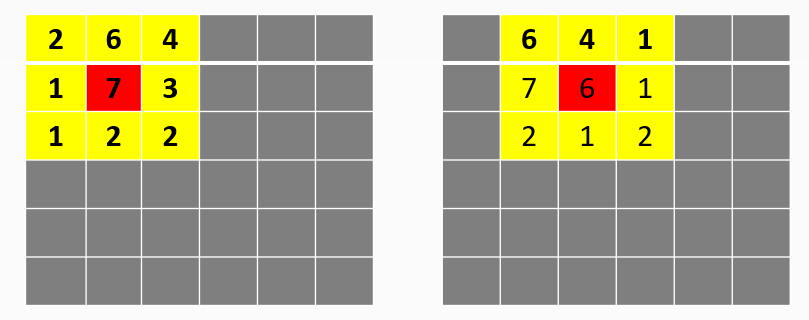数学公式如下：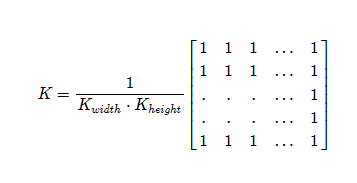如上图1中心点数经过均值模糊后为（2+6+4+1+7+3+1+2+2）/ 9 = 3

API函数：

void cv::blur ( InputArray  src,
OutputArray  dst,
Size  	ksize,
Point  	anchor = Point(-1,-1),
int  	borderType = BORDER_DEFAULT
)


作用：均值滤波，图像均值模糊处理

输入参数 参数定义
src 输入图像
dst 输出图像
ksize 模糊核大小
anchor 锚点，（-1，-1）为中心点
borderType 边框模式用于推断图像外部的像素

## 高斯滤波

高斯滤波对图像处理的过程与均值滤波类似，但高斯滤波符合正态分布，在计算矩阵值时，不再通过平均值的方法计算像素点，而是通过正态分布的方法进行计算，其本质上是对掩模矩阵中的所有像素值进行加权平均，虽然高斯滤波克服了部分丢失边缘像素的缺陷，但由于高斯滤波在计算时，不考虑像素值的不同，因此无法完全避免。

数学公式如下：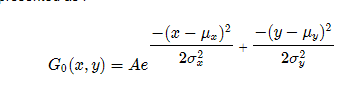API函数：

void cv::GaussianBlur ( InputArray  	src,
OutputArray  	dst,
Size  	ksize,
double  	sigmaX,
double  	sigmaY = 0,
int  	borderType = BORDER_DEFAULT
)


作用：高斯滤波，图像高斯模糊处理

输入参数 参数定义
src 输入图像
dst 输出图像
ksize 模糊核大小，但它们必须是正的和奇数的
sigmaX X方向上的高斯核标准差
sigmaY Y方向上的高斯核标准差
borderType 边框模式用于推断图像外部的像素

## 中值滤波

高斯滤波对图像处理的过程与均值滤波类似，在计算矩阵值时，不再通过平均值的方法计算像素点，而是通过取中值的方法进行计算。该方法对于椒盐噪声（极大值、极小值）有很好的抑制作用，但对边缘化像素处理仍然不够完善。
如果一个3X3矩阵中为2、6、4、1、7、3、1、2、2，经过从小到大的排列1、1、2、2、2、3、4、6、7，则中值则取2，像素点赋值为2.

API函数：

void cv::medianBlur (   InputArray  	src,
OutputArray  	dst,
int  	ksize
)


作用：中值滤波，图像中值模糊处理

输入参数 参数定义
src 输入图像
dst 输出图像
ksize 孔径线性尺寸;它必须是奇数并且大于1

## 双边滤波

高斯双边滤波为了完善高斯滤波的缺点，是边缘保留的滤波方法，避免了边缘信息丢失，保留了图像轮廓的不变。相对于只考虑空间核的高斯滤波，双边滤波加入了值域核，从而将像素值相差过大的值进行保留处理，其多数用于美艳照片上。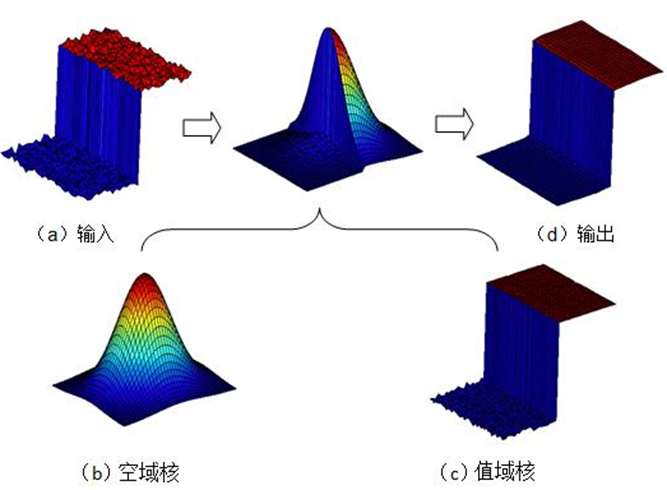API函数：

void cv::bilateralFilter (  InputArray  	src,
OutputArray  	dst,
int  	d,
double  	sigmaColor,
double  	sigmaSpace,
int  	borderType = BORDER_DEFAULT
)


作用：双边滤波，图像双边模糊处理

输入参数 参数定义
src 输入图像
dst 输出图像
d 用于滤波的每个像素邻域的直径，直径内的所有像素均会被纳入计算。如果它是非正数，则从sigmaSpace计算
sigmaColor 决定多少差值内的像素会被计算
sigmaSpace 如果d的值大于0则无效，否则会用来计算d的值
borderType 边框模式用于推断图像外部的像素

## 示例

以均值滤波、高斯滤波、中值滤波、双边滤波采用5*5左右核对同一张图片进行模糊。

#include "mainwindow.h"
#include "ui_mainwindow.h"
#include <opencv2/opencv.hpp>
#include <QtDebug>

using namespace cv;

MainWindow::MainWindow(QWidget *parent) :
QMainWindow(parent),
ui(new Ui::MainWindow)
{
ui->setupUi(this);
Mat dstBlur;
Mat dstGaussianBlur;
Mat dstmedianBlur;
Mat dstbilateralFilter;
if(src.empty()){
return ;
}
namedWindow("srcImage",CV_WINDOW_AUTOSIZE);
namedWindow("均值滤波",CV_WINDOW_AUTOSIZE);
namedWindow("高斯滤波",CV_WINDOW_AUTOSIZE);
namedWindow("中值滤波",CV_WINDOW_AUTOSIZE);
namedWindow("双边滤波",CV_WINDOW_AUTOSIZE);
//均值滤波
blur(src,dstBlur,Size(5,5));
//高斯滤波
GaussianBlur(src,dstGaussianBlur,Size(5,5),11,11);
//中值滤波
medianBlur(src,dstmedianBlur,5);
//双边滤波
bilateralFilter(src,dstbilateralFilter,5,100,3);

imshow("srcImage",src);
imshow("均值滤波",dstBlur);
imshow("高斯滤波",dstGaussianBlur);
imshow("中值滤波",dstmedianBlur);
imshow("双边滤波",dstbilateralFilter);
waitKey(0);


原图：均值滤波：高斯滤波：中值滤波：双边滤波：图像处理 模糊处理作用 相关内容

2015-12-17 16:49:24 liushaofang 阅读数 568
• ###### MATLAB图像处理

全面系统的学习MATLAB在图像处理中的应用

20258 人正在学习 去看看 魏伟

对图像进行高斯模糊的处理类，实际应用效果不错！！！

package util;

import android.annotation.SuppressLint;
import android.content.Context;
import android.graphics.Bitmap;
import android.os.Build.VERSION;
import android.renderscript.Allocation;
import android.renderscript.Allocation.MipmapControl;
import android.renderscript.Element;
import android.renderscript.RenderScript;
import android.renderscript.ScriptIntrinsicBlur;
import java.lang.reflect.Array;

public class Blur
{
private static final String TAG = "Blur";

@SuppressLint({"NewApi"})
public static Bitmap fastblur(Context paramContext, Bitmap paramBitmap, int paramInt)
{
if (Build.VERSION.SDK_INT > 16)
{
Bitmap localBitmap2 = paramBitmap.copy(paramBitmap.getConfig(), true);
RenderScript localRenderScript = RenderScript.create(paramContext);
Allocation localAllocation1 = Allocation.createFromBitmap(localRenderScript, paramBitmap, Allocation.MipmapControl.MIPMAP_NONE, 1);
Allocation localAllocation2 = Allocation.createTyped(localRenderScript, localAllocation1.getType());
ScriptIntrinsicBlur localScriptIntrinsicBlur = ScriptIntrinsicBlur.create(localRenderScript, Element.U8_4(localRenderScript));
localScriptIntrinsicBlur.setInput(localAllocation1);
localScriptIntrinsicBlur.forEach(localAllocation2);
localAllocation2.copyTo(localBitmap2);
return localBitmap2;
}
Bitmap localBitmap1 = paramBitmap.copy(paramBitmap.getConfig(), true);
if (paramInt < 1)
return null;
int i = localBitmap1.getWidth();
int j = localBitmap1.getHeight();
int[] arrayOfInt1 = new int[i * j];
localBitmap1.getPixels(arrayOfInt1, 0, i, 0, 0, i, j);
int k = i - 1;
int m = j - 1;
int n = i * j;
int i1 = 1 + (paramInt + paramInt);
int[] arrayOfInt2 = new int[n];
int[] arrayOfInt3 = new int[n];
int[] arrayOfInt4 = new int[n];
int[] arrayOfInt5 = new int[Math.max(i, j)];
int i2 = i1 + 1 >> 1;
int i3 = i2 * i2;
int[] arrayOfInt6 = new int[i3 * 256];
for (int i4 = 0; ; i4++)
{
int i5 = i3 * 256;
if (i4 >= i5)
break;
arrayOfInt6[i4] = (i4 / i3);
}
int i6 = 0;
int i7 = 0;
int[] arrayOfInt7 = { i1, 3 };
int[][] arrayOfInt = (int[][])Array.newInstance(Integer.TYPE, arrayOfInt7);
int i8 = paramInt + 1;
for (int i9 = 0; i9 < j; i9++)
{
int i37 = 0;
int i38 = 0;
int i39 = 0;
int i40 = 0;
int i41 = 0;
int i42 = 0;
int i43 = 0;
int i44 = 0;
int i45 = 0;
int i46 = -paramInt;
if (i46 <= paramInt)
{
int i59 = arrayOfInt1[(i6 + Math.min(k, Math.max(i46, 0)))];
int[] arrayOfInt13 = arrayOfInt[(i46 + paramInt)];
arrayOfInt13 = ((0xFF0000 & i59) >> 16);
arrayOfInt13 = ((0xFF00 & i59) >> 8);
arrayOfInt13 = (i59 & 0xFF);
int i60 = i8 - Math.abs(i46);
i39 += i60 * arrayOfInt13;
i38 += i60 * arrayOfInt13;
i37 += i60 * arrayOfInt13;
if (i46 > 0)
{
i45 += arrayOfInt13;
i44 += arrayOfInt13;
i43 += arrayOfInt13;
}
while (true)
{
i46++;
break;
i42 += arrayOfInt13;
i41 += arrayOfInt13;
i40 += arrayOfInt13;
}
}
int i47 = paramInt;
for (int i48 = 0; i48 < i; i48++)
{
arrayOfInt2[i6] = arrayOfInt6[i39];
arrayOfInt3[i6] = arrayOfInt6[i38];
arrayOfInt4[i6] = arrayOfInt6[i37];
int i49 = i39 - i42;
int i50 = i38 - i41;
int i51 = i37 - i40;
int[] arrayOfInt11 = arrayOfInt[((i1 + (i47 - paramInt)) % i1)];
int i52 = i42 - arrayOfInt11;
int i53 = i41 - arrayOfInt11;
int i54 = i40 - arrayOfInt11;
if (i9 == 0)
arrayOfInt5[i48] = Math.min(1 + (i48 + paramInt), k);
int i55 = arrayOfInt1[(i7 + arrayOfInt5[i48])];
arrayOfInt11 = ((0xFF0000 & i55) >> 16);
arrayOfInt11 = ((0xFF00 & i55) >> 8);
arrayOfInt11 = (i55 & 0xFF);
int i56 = i45 + arrayOfInt11;
int i57 = i44 + arrayOfInt11;
int i58 = i43 + arrayOfInt11;
i39 = i49 + i56;
i38 = i50 + i57;
i37 = i51 + i58;
i47 = (i47 + 1) % i1;
int[] arrayOfInt12 = arrayOfInt[(i47 % i1)];
i42 = i52 + arrayOfInt12;
i41 = i53 + arrayOfInt12;
i40 = i54 + arrayOfInt12;
i45 = i56 - arrayOfInt12;
i44 = i57 - arrayOfInt12;
i43 = i58 - arrayOfInt12;
i6++;
}
i7 += i;
}
for (int i10 = 0; i10 < i; i10++)
{
int i11 = 0;
int i12 = 0;
int i13 = 0;
int i14 = 0;
int i15 = 0;
int i16 = 0;
int i17 = 0;
int i18 = 0;
int i19 = 0;
int i20 = i * -paramInt;
int i21 = -paramInt;
if (i21 <= paramInt)
{
int i35 = i10 + Math.max(0, i20);
int[] arrayOfInt10 = arrayOfInt[(i21 + paramInt)];
arrayOfInt10 = arrayOfInt2[i35];
arrayOfInt10 = arrayOfInt3[i35];
arrayOfInt10 = arrayOfInt4[i35];
int i36 = i8 - Math.abs(i21);
i13 += i36 * arrayOfInt2[i35];
i12 += i36 * arrayOfInt3[i35];
i11 += i36 * arrayOfInt4[i35];
if (i21 > 0)
{
i19 += arrayOfInt10;
i18 += arrayOfInt10;
i17 += arrayOfInt10;
}
while (true)
{
if (i21 < m)
i20 += i;
i21++;
break;
i16 += arrayOfInt10;
i15 += arrayOfInt10;
i14 += arrayOfInt10;
}
}
int i22 = i10;
int i23 = paramInt;
for (int i24 = 0; i24 < j; i24++)
{
arrayOfInt1[i22] = (0xFF000000 & arrayOfInt1[i22] | arrayOfInt6[i13] << 16 | arrayOfInt6[i12] << 8 | arrayOfInt6[i11]);
int i25 = i13 - i16;
int i26 = i12 - i15;
int i27 = i11 - i14;
int[] arrayOfInt8 = arrayOfInt[((i1 + (i23 - paramInt)) % i1)];
int i28 = i16 - arrayOfInt8;
int i29 = i15 - arrayOfInt8;
int i30 = i14 - arrayOfInt8;
if (i10 == 0)
arrayOfInt5[i24] = (i * Math.min(i24 + i8, m));
int i31 = i10 + arrayOfInt5[i24];
arrayOfInt8 = arrayOfInt2[i31];
arrayOfInt8 = arrayOfInt3[i31];
arrayOfInt8 = arrayOfInt4[i31];
int i32 = i19 + arrayOfInt8;
int i33 = i18 + arrayOfInt8;
int i34 = i17 + arrayOfInt8;
i13 = i25 + i32;
i12 = i26 + i33;
i11 = i27 + i34;
i23 = (i23 + 1) % i1;
int[] arrayOfInt9 = arrayOfInt[i23];
i16 = i28 + arrayOfInt9;
i15 = i29 + arrayOfInt9;
i14 = i30 + arrayOfInt9;
i19 = i32 - arrayOfInt9;
i18 = i33 - arrayOfInt9;
i17 = i34 - arrayOfInt9;
i22 += i;
}
}
localBitmap1.setPixels(arrayOfInt1, 0, i, 0, 0, i, j);
return localBitmap1;
}
}

图像处理 模糊处理作用 相关内容

2018-11-26 09:46:30 qq_41877629 阅读数 475
• ###### MATLAB图像处理

全面系统的学习MATLAB在图像处理中的应用

20258 人正在学习 去看看 魏伟

### 浅谈图像去模糊化

电子科技大学格拉斯哥学院2017级姚振球

# 摘要

新生研讨课上曾兵院长为我们介绍的图像处理技术让我产生了浓厚的兴趣，于是查阅了相关资料，对图像处理技术有了更为深刻的了解。同时，随着数学与计算机科学的飞速发展，以及军事、医学、工业等各个领域对图像处理要求的不断提高，该领域已经表现出其长远的发展前景与研究意义。本文侧重于讨论图像增强与复原。将从图像模糊原因的分析、模糊图像处理入手，介绍一种最为基础的图像去模糊化算法，在文章末尾，还会提及一些目前较前沿的图像去模糊化的方法与原理。

# 图像模糊原因分析

其实造成图像模糊的原因很多，聚焦不准、光学系统的像差、成像过程中的相对运动、大气湍流效应、低光照、环境随机噪声等都会导致图像模糊。另外图像的编解码、传输过程都可能导致图像的进一步模糊。其中最为主要的原因有以下几大方面。

1、系统自身因素

图像在采集、传输、存储、显示的过程中，都有或多或少的信息损失，也就导致图像失真变模糊。这也很好理解，举一个例子，当我们用一台照相机去拍摄另一台照相机中的照片，这样反复若干次，就会发现照片越来越模糊不清。其次，曝光量和曝光时间也是一个需要考虑的因素。

2、自然环境

除系统本身因素以外，自然环境也会影响图片的质量。刮风、下雨、下雪、大雾等天气都会导致图像质量下降或者模糊不清。还有背光、逆光等光线不足的地方，摄像机的感应器成像就会产生很多噪音，这些噪音会影响图像清晰度。

3、人为环境

视频压缩算法、运动目标高速运动导致的运动模糊等

# 模糊图像常用的处理方法

前面也已经提到，造成图像模糊的原因有很多，要取得比较好的处理效果，不同原因导致的模糊往往需要不同的处理方法。从技术方面来说，模糊图像处理方法主要分为三大类：图像增强、图像复原和超分辨率重构。

1、图像增强

简单来说，图像增强就是为了改善图像的视觉效果，有目的性的强调局部特征信息。它可以是一个失真的过程。

图像增强可分为两大类：频率域法和空间域法。前者吧图像可看成是一种二维信号，对其进行基于二维傅里叶变换的信号增强。采用低通滤波法，可去除途中的噪音；采用高通滤波法，则可以增强边缘等高频信号，使模糊的图像变得清晰。具有代表性的空间域算法有局部求平均值算法和中值滤波法，他们可用于去除或减弱噪音。

2、图像复原

在成像过程中，由于成像系统各种因素的影响，可能使获得的图像不是真实景物的完善影像。图像在形成、传播和保存过程中使图像质量下降的过程，称之为退化。图像复原就是重建退化的图像，使其最大限度恢复景物原貌的处理。图喜爱那个复原只能尽量使图像接近其原始图像，但是由于噪音干扰等因素，很难精准还原。

还需要解释的是图像增强与复原的区别。图像增强的目的是消除噪音，显示出那些被模糊的细节，不考虑图像质量下降的，而图像复原是利用退化现象的某些先验知识，建立退化现象的数学模型，再根据模型进行逆运算，来恢复之前的图像。

3、超分辨率重构

就是通过一系列低分辨率的图像来得到一副高分辨率图像过程，目前有很多方法能实现超分辨率重建，包括“稀疏编码法（Sparse Coding）”、“贝叶斯方法”等等

# 匀速直线运动模糊的退化模型分析

在所有运动模糊中，匀速运动所导致的模糊是最有普遍性的。因为变速运动可以看成是极短时间内匀速运动的叠加。【1】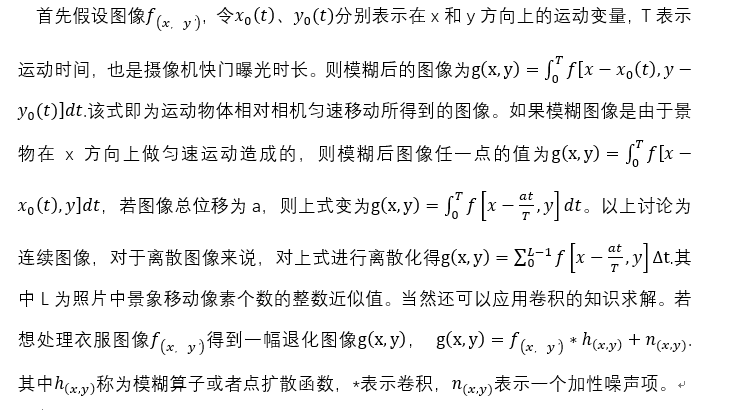# 较前沿的图像去模糊化的方法

简单的图像模糊可以看作是模糊核与原始图像进行了卷积，因此模糊核估计是图像去模糊的一个重要部分。如果模糊核已知，那么算法就退化为Non-blind image deconvolution。

Jiaya Jia的方法主要是针对一张已经有运动模糊的图像，算法分为模糊核估计以及图像恢复两个步骤，这两步构成了一个迭代优化框架。首先估计模糊核，然后对图像恢复，然后再重新估计模糊核，恢复图像，最终知道收敛。他另一个主要贡献在于分析了造成图像模糊的多种因素，进而提出了估计模糊核更好的概率模型。

图像处理 模糊处理作用 相关内容

2018-05-15 14:09:16 Blaze_bxh 阅读数 1802
• ###### MATLAB图像处理

全面系统的学习MATLAB在图像处理中的应用

20258 人正在学习 去看看 魏伟

学习目标：

1. 使用不同的低通滤波器模糊图像；

2. 对图像使用定制化滤波器；

## 2D卷积

低通滤波器用于去除噪音信息，模糊图像，高通滤波器用于寻找图像中的边缘信息。

Opencv提供的接口函数cv2.filter2D()来对图像做卷积。我们定义一个5x5的均值滤波器核，如下：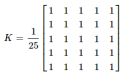操作方法：将该滤波器置于一个像素之上，在该滤波器的范围内，所有像素相加，得到均值，用均值替换中间的像素值。该操作作用于图像中的所有像素后，即完成均值滤波。

## 图像模糊（图像平滑）

通过对图像做低通滤波器的卷积操作，实现图像模糊，从而去除噪声信息。实际上，就是从图像中去除了高频信息（如噪点，边缘）。

Opencv提供了四种图像模糊的技术。

### 1. Averaging

使用标准化的滤波器对图像做卷积，简单地将卷积核区域内的所有像素取均值，并且用均值替换卷积核中心位置对应的像素值。

Opencv 接口：cv2.blur(), cv2.boxFilter(),只需要指定卷积核的宽高即可。

### 2. Gaussian blurring

与上节不同，此处使用高斯核。需要指定核的宽高（正数，奇数），指定xy方向上的偏差，sigmaXsigmaY

高斯模糊可以高效去除图像中的高斯噪音，降低细节层次。

高斯滤波其实就是用高斯分布的方式，生成滤波器中各个位置的权重，再用该滤波器对图像进行卷积操作。

### 3. Median Blurring

取核区域内的所有像素的中间值，用该值替换核中心的像素值。这种滤波方式，可以高效处理椒盐噪声。在前面介绍的滤波方式中，中间像素的替换值可能是新产生的，而中值滤波，中间值只是被图像中的某个像素值替换。这种方式可以有效减少噪声。

### 4. Bilateral Filtering

双边滤波可以在保留边缘信息的同时，高效去除噪声。但是该操作相比其他滤波器，速度更慢。

高斯滤波器基于位置对各像素进行高斯分布的加权处理，但是他没有考虑到像素之间是否有相同的强度，没有考虑像素是否是边缘像素，所以高斯模糊会将边缘一起模糊掉。

双边滤波器在空间上也使用了高斯滤波器，同时又加了一个高斯滤波器是像素差分函数。空间的高斯函数确保临近像素间的模糊处理，同时，强度差分的高斯函数确保只有和中心像素的强度类似的像素才可以被模糊，因此可以保留边缘信息。

图像处理 模糊处理作用 相关内容

阅读数 7810

博文 来自： maozefa

阅读数 4394

博文 来自： maozefa

阅读数 4083

博文 来自： smtctc

阅读数 11682

博文 来自： maozefa

阅读数 241

博文 来自： qq_28061765
没有更多推荐了，返回首页